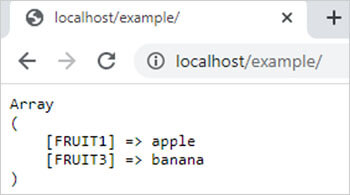PHP array_udiff_assoc() Function || PHP Array Functions

# PHP array_udiff_assoc() function

0 1272

array_udiff_assoc() function of PHP is used to return the difference of two or more arrays by comparing the keys and values of arrays with the help of a user-defined function or the callback function.

It is an inbuilt function of PHP.

Syntax:

array_udiff_assoc(\$array1, \$array2, ........, \$arrayN, user_function);

here,

\$array1, \$array2 and \$arrayN are php arrays and user_function is a user define function which used to calculate the difference between arrays and return the unique elements of first array.

Example 1:

```<?php
function user_function(\$x,\$y)
{
if (\$x===\$y)
{
return 0;
}
return (\$x>\$y)?1:-1;
}
\$arr1=array("FRUIT1"=>"apple","FRUIT2"=>"guava","FRUIT3"=>"banana");
\$arr2=array("FRUIT4"=>"apple","FRUIT2"=>"guava","FRUIT5"=>"fig");
\$result=array_udiff_assoc(\$arr1,\$arr2,"user_function");
echo "<pre>";
print_r(\$result);
?>```

Output:Share: